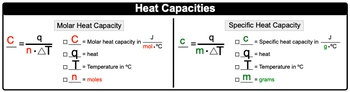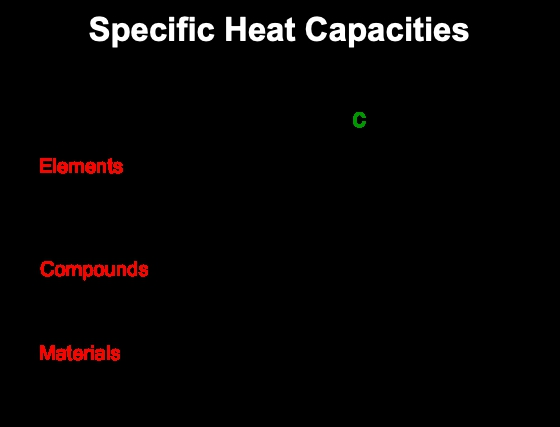Start typing, then use the up and down arrows to select an option from the list.## General Chemistry

Learn the toughest concepts covered in Chemistry with step-by-step video tutorials and practice problems by world-class tutors

8. Thermochemistry

Heat Capacity is the amount of heat required to change the temperature of a substance.

Understanding Heat Capacity
1
concept

## Heat Capacity31s
Play a video:
So when we talk about heat capacity, we're talking about the application of heat toe a substance we're going to say as we heat an object, its temperature increases because heat is directly proportional to its temperature change. The more heat I apply to something, the greater the temperature will change will be so here we're toe illustrate that we say that Q being directly proportional to Delta T. So just remember that is the relationship between heat and temperature.2
example

## Heat Capacity Example 133s
Play a video:
here, it says, if the temperature of the water bath goals from 25 Calvin to 50 Kelvin, what could be said about the amount of heat? So remember we said that heat, which is Q. Is directly proportional to change in temperature. Here are temperatures going from 25 Calvin to 50 Kelvin, so it is being doubled in terms of Kelvin. And since there directly proportional, what happens to one happens to the other. With our temperature doubling, that would mean that my heat would also have to double. This means that option a would be are correct answer.
3
concept

## Heat Capacity2m
Play a video:
now he capacity, which uses capital C, is the amount of heat required to change the temperature of awaited substance. The more heat that's applied to a substance, the greater its temperature change. Now it could be looked at also in terms of specific he capacity and Mueller he capacity with specifically capacity. We lose. We use lower case C, and it is the amount of heat required to change the temperature of 1 g of a substance by one degree. That degree could be either in Kelvin or Degrees Celsius. Here, Mueller he capacity is just like he capacity in terms that it be being a capital seat. But here, with Mueller, he capacity. It's the amount of heat required to change the temperature off one mole of a substance by one degree, either in Kelvin or degrees Celsius. Okay, so think of Mueller. He capacity as being a little bit more, um, focused in terms of the way we look at he capacity in terms of one mole of a substance. Now we're going to say here that when it comes to a Moeller heat capacity, which is capital C, it equals Q over N Times Delta T so Capital C equals R. Mueller. He capacity in jewels over moles times degrees Celsius or in Kelvin Q represents heat. T equals temperature in degrees Celsius. But what we need to realize here is that whatever the units that the Mueller he capacity uses for temperature temperature should match it. Okay, so if this happened to be in Kelvin in, temperature should be in Kelvin and an end is equal to our moles with our specific heat capacity. Uses lower case C in equals Q. Over M times Delta T Here, Lower case C is our specifically capacity in jewels over grants times degrees Celsius or Kelvin que again his heat temperature again can be in Celsius or in Calvin to determine which one to use. You look at the units for your specifically capacity and make sure they match and then lower case. And here is just grams of our substance. So just remember the difference between Mueller he capacity and specifically capacity4
example

## Heat Capacity Example 21m
Play a video:
here. The example says 15.7 g silver raises its temperature by 17.2 degrees Celsius when it absorbs 6845.5 jewels. What is its Mueller? He capacity? So Mueller Heat capacity uses Capital C. It's equal to heat, which is Q divided by moles. End times change in temperature In the question. It says that we're absorbing this much energy. That means that that's a positive. Q. So that's positive. 6145 5 jewels. Next, we need moles, and we already have the change in temperature. They said that the temperature was risen by 17.2 degrees Celsius, so that's already are change in temperature. We need moles. We have here 15.7 g of silver, which is a G. We have to change that. Two moles. So one mole of Silver Ways 107. g according to the periodic table. So that comes out to be 0. Moles of silver. Take those moles and plug it in. So when we do that, that's going to give me my Mueller. He capacity as 2734. Jules Over Moles times, degrees Celsius. If we look at our values, this has three sig figs and this has three sig figs. So I could change this to 2.73 times 10 to the three Jules over moles times degrees Celsius. So that would be the multi capacity for silver under these conditions.
5
concept

## Heat Capacity44s
Play a video:
now by rearranging the specifically capacity given above, we can solve for the amount of heat released or absorbed. Here are new specific heat capacity. Formula becomes Q equals. M times C Times Delta T For all of you pre med track students We usually say that this is equal to Q equals M. Cat, and we know that the cat is an important exam before you matriculated into medical school. So use that to help you remember it. So Q equals M cat is our new formula. Help us determine and relate the specific e capacity to the amount of heat absorbed or released in a chemical reaction.6
example

## Heat Capacity Example 32m
Play a video:
here, it says how much heat in killing jewels is released when 120 g of water goes from 90 degrees Celsius to 45 degrees Celsius. The specifically capacity of water is given. Ask 4.184 jewels over Graham's times degrees Celsius. So we need to determine the heat, which is cute. They're giving us here the mass of the water, which is M. We have our change in temperature here, and we have our specific heat capacity, which is lower case C. So Q equals M capped. Plug in the grams of water given to us plug in the specific heat capacity that's given to us. And remember the units for the specific heat capacity dictate the units for temperature. Since this is in Celsius, it's good to have our temperatures in Celsius as well. Delta T is final minus initial. So when we do all that Celsius, cancel out, grams can slumped and we'll have jewels of heat involved. When we plugged that in, we're going to get here. Negative. 22593.6 jewels. But here we want the answer in Kila jewels. So one killer, Jewell is equal to tend to the three jewels. Yeah, so here, Jules, cancel out and we'll have killer jewels at the end. So that comes out to negative. 22 point 5936 Killer jewels here. This has four sig figs, one sigfig to sig figs, specifically capacity. We could use it, since it's given to us as a value, but we don't have to. Here. We could just go with one sigfig, but I feel like that's too much rounding. So let's just go with two sig figs based on 45 So it's give me negative 23. Roughly killing joules of heat are released. So here, using Q equals M cat were ableto isolate the heat that's involved in the releasing by the water molecule going from 90 degrees Celsius to 45 degrees Celsius.
7
Problem

A sample of copper absorbs 3.53 kJ of heat, which increases the temperature by 25 ºC, determine the mass (in kg) of the copper sample if the specific heat capacity of copper is 0.385 J / g ºC.

8
Problem

Based on their given specific heat capacities which compound would show the greatest temperature change upon absorbing 25.0 J of heat?9
Problem

50.00 g of heated metal ore is placed into an insulated beaker containing 822.5 g of water. Once the metal heats up the final temperature of the water is 32.08 ºC. If the metal gains 14.55 kJ of energy, what is the initial temperature of the water? The specific heat capacity of copper is 4.184 J / g ºC.